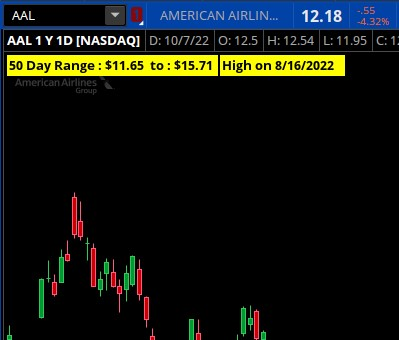# Change defined lookback to an input

#### stormy77

##### Member
Good afternoon,
I'm using the script below to show price range of a specific period as a label and a high price label during that period. I'd like to be able to convert this to a script so I can specify the period value in the interface, instead of having to create a new script for each value. So If I wanted to use a 60 day range, all I would need to do is type in 60 in the interface. Hope I was able to explain.

Here's the existing script I use:

Code:
``````def hi14 = Highest(high(period = AggregationPeriod.Day),14);
def low14 = Lowest(low(period = AggregationPeriod.Day),14);
def LastBar = HighestAll(if !IsNaN(close) then BarNumber() else Double.NaN);
def LastHigh = HighestAll(if BarNumber() == LastBar then Highest(high(period = AggregationPeriod.Day),14) else Double.NaN);
def Day; def Month; def Year;
if High == LastHigh{
Day = GetDayOfMonth(getyYYYMMDD());
Month = getmonth();
Year = getYear();
} else {
Day = Day;
Month = Month;
Year = Year;
}

addlabel(1, " 14 Day Range : \$" + Round(low14, 2) + "  to : \$" + Round(hi14, 2) +" ", color.YELLOW);
Addlabel(yes, "High"+" on " + month + "/" + day + "/" + asprice(year)+"   ",color.YELLOW);
#spacer
input Spacer = yes;
#spacer labelSolution
Good afternoon,
I'm using the script below to show price range of a specific period as a label and a high price label during that period. I'd like to be able to convert this to a script so I can specify the period value in the interface, instead of having to create a new script for each value. So If I wanted to use a 60 day range, all I would need to do is type in 60 in the interface. Hope I was able to explain.

Here's the existing script I use:

https://i.postimg.cc/43Z7Ddzw/snip.jpg
Code:
``````def hi14 = Highest(high(period = AggregationPeriod.Day),14);
def low14 = Lowest(low(period = AggregationPeriod.Day),14);
def LastBar = HighestAll(if !IsNaN(close) then BarNumber() else Double.NaN);
def LastHigh = HighestAll(if BarNumber() ==...``````

#### SleepyZ

##### Well-known member
VIP
Good afternoon,
I'm using the script below to show price range of a specific period as a label and a high price label during that period. I'd like to be able to convert this to a script so I can specify the period value in the interface, instead of having to create a new script for each value. So If I wanted to use a 60 day range, all I would need to do is type in 60 in the interface. Hope I was able to explain.

Here's the existing script I use:

https://i.postimg.cc/43Z7Ddzw/snip.jpg
Code:
``````def hi14 = Highest(high(period = AggregationPeriod.Day),14);
def low14 = Lowest(low(period = AggregationPeriod.Day),14);
def LastBar = HighestAll(if !IsNaN(close) then BarNumber() else Double.NaN);
def LastHigh = HighestAll(if BarNumber() == LastBar then Highest(high(period = AggregationPeriod.Day),14) else Double.NaN);
def Day; def Month; def Year;
if High == LastHigh{
Day = GetDayOfMonth(getyYYYMMDD());
Month = getmonth();
Year = getYear();
} else {
Day = Day;
Month = Month;
Year = Year;
}

addlabel(1, " 14 Day Range : \$" + Round(low14, 2) + "  to : \$" + Round(hi14, 2) +" ", color.YELLOW);
Addlabel(yes, "High"+" on " + month + "/" + day + "/" + asprice(year)+"   ",color.YELLOW);
#spacer
input Spacer = yes;
#spacer labelThis should helpRuby:
``````input range = 14;
def hirange = Highest(high(period = AggregationPeriod.DAY), range);
def lowrange = Lowest(low(period = AggregationPeriod.DAY), range);
def LastBar = HighestAll(if !IsNaN(close) then BarNumber() else Double.NaN);
def LastHigh = HighestAll(if BarNumber() == LastBar then Highest(high(period = AggregationPeriod.DAY), range) else Double.NaN);
def Day;
def Month;
def Year;
if high == LastHigh {
Day = GetDayOfMonth(GetYYYYMMDD());
Month = GetMonth();
Year = GetYear();
} else {
Day = Day;
Month = Month;
Year = Year;
}

AddLabel(1, range + " Day Range : \$" + Round(lowrange, 2) + "  to : \$" + Round(hirange, 2) + " ", Color.YELLOW);
AddLabel(yes, "High" + " on " + Month + "/" + Day + "/" + AsPrice(Year) + "   ", Color.YELLOW);
#spacer
input Spacer = yes;
#spacer label

#### stormy77

##### Member
Thank you very much. this should help me understand how to modify other types of scripts as well.

•SleepyZ## The Market Trading Game Changer

Join 2,500+ subscribers inside the useThinkScript VIP Membership Club
• Exclusive indicators
• Proven strategies & setups
• Private Discord community
• Exclusive members-only content
• 1 full year of unlimited support

What is useThinkScript?

useThinkScript is the #1 community of stock market investors using indicators and other tools to power their trading strategies. Traders of all skill levels use our forums to learn about scripting and indicators, help each other, and discover new ways to gain an edge in the markets.

How do I get started?

We get it. Our forum can be intimidating, if not overwhelming. With thousands of topics, tens of thousands of posts, our community has created an incredibly deep knowledge base for stock traders. No one can ever exhaust every resource provided on our site.

If you are new, or just looking for guidance, here are some helpful links to get you started.

What are the benefits of VIP Membership?